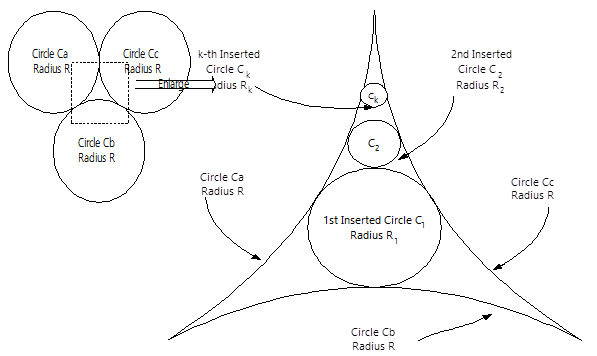×

# 计蒜客

1. 题库
2. Finding the Radius for an Inserted Circle
3. 问答
• 32.18%
• 131072K

Three circles $C_{a}$, $C_{b}$, and $C_{c}$, all with radius $R$ and tangent to each other, are located in two-dimensional space as shown in Figure $1$. A smaller circle $C_{1}$ with radius $R_{1}$ ($R_{1}<R$) is then inserted into the blank area bounded by $C_{a}$, $C_{b}$, and $C_{c}$ so that $C_{1}$ is tangent to the three outer circles, $C_{a}$, $C_{b}$, and $C_{c}$. Now, we keep inserting a number of smaller and smaller circles $C_{k}\ (2 \leq k \leq N)$ with the corresponding radius $R_{k}$ into the blank area bounded by $C_{a}$, $C_{c}$ and $C_{k-1}$ $(2 \leq k \leq N)$, so that every time when the insertion occurs, the inserted circle $C_{k}$ is always tangent to the three outer circles $C_{a}$, $C_{c}$ and $C_{k-1}$, as shown in Figure $1$Figure 1.

(Left) Inserting a smaller circle $C_{1}$ into a blank area bounded by the circle $C_{a}$, $C_{b}$ and $C_{c}$.

(Right) An enlarged view of inserting a smaller and smaller circle $C_{k}$ into a blank area bounded by $C_{a}$, $C_{c}$ and $C_{k-1}$ ($2 \leq k \leq N$), so that the inserted circle $C_{k}$ is always tangent to the three outer circles, $C_{a}$, $C_{c}$, and $C_{k-1}$.

Now, given the parameters $R$ and $k$, please write a program to calculate the value of $R_{k}$, i.e., the radius of the $k-th$ inserted circle. Please note that since the value of $R_k$ may not be an integer, you only need to report the integer part of $R_{k}$. For example, if you find that $R_{k}$ = $1259.8998$ for some $k$, then the answer you should report is $1259$.

Another example, if $R_{k}$ = $39.1029$ for some $k$, then the answer you should report is $39$.

Assume that the total number of the inserted circles is no more than $10$, i.e., $N \leq 10$. Furthermore, you may assume $\pi = 3.14159$. The range of each parameter is as below:

$1 \leq k \leq N$, and $10^{4} \leq R \leq 10^{7}$.

### Input Format

Contains $l + 3$ lines.

Line $1$: $l$ ----------------- the number of test cases, $l$ is an integer.

Line $2$: $R$ ---------------- $R$ is a an integer followed by a decimal point,then followed by a digit.

Line $3$: $k$ ---------------- test case #$1$, $k$ is an integer.

$\ldots$

Line $i+2$: $k$ ----------------- test case # $i$.

$\ldots$

Line $l +2$: $k$ ------------ test case #$l$.

Line $l + 3$: $-1$ ---------- a constant $-1$ representing the end of the input file.

### Output Format

Contains $l$ lines.

Line $1$: $k$ $R_{k}$ ----------------output for the value of $k$ and $R_{k}$ at the test case #$1$, each of which should be separated by a blank.

$\ldots$

Line $i$: $k$ $R_{k}$ ----------------output for $k$ and the value of $R_{k}$ at the test case # $i$, each of which should be separated by a blank.

Line $l$: $k$ $R_{k}$ ----------------output for $k$ and the value of$R_{k}$ at the test case # $l$, each of which should be separated by a blank.

#### 样例输入

1
152973.6
1
-1

#### 样例输出

1 23665

• main.c